JEE  >  Competition Level Test: Continuity And Differentiability- 2

# Competition Level Test: Continuity And Differentiability- 2

Test Description

## 30 Questions MCQ Test Mathematics (Maths) Class 12 | Competition Level Test: Continuity And Differentiability- 2

Competition Level Test: Continuity And Differentiability- 2 for JEE 2023 is part of Mathematics (Maths) Class 12 preparation. The Competition Level Test: Continuity And Differentiability- 2 questions and answers have been prepared according to the JEE exam syllabus.The Competition Level Test: Continuity And Differentiability- 2 MCQs are made for JEE 2023 Exam. Find important definitions, questions, notes, meanings, examples, exercises, MCQs and online tests for Competition Level Test: Continuity And Differentiability- 2 below.
Solutions of Competition Level Test: Continuity And Differentiability- 2 questions in English are available as part of our Mathematics (Maths) Class 12 for JEE & Competition Level Test: Continuity And Differentiability- 2 solutions in Hindi for Mathematics (Maths) Class 12 course. Download more important topics, notes, lectures and mock test series for JEE Exam by signing up for free. Attempt Competition Level Test: Continuity And Differentiability- 2 | 30 questions in 60 minutes | Mock test for JEE preparation | Free important questions MCQ to study Mathematics (Maths) Class 12 for JEE Exam | Download free PDF with solutions
 1 Crore+ students have signed up on EduRev. Have you?
Competition Level Test: Continuity And Differentiability- 2 - Question 1

### The function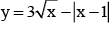is continuous at

Detailed Solution for Competition Level Test: Continuity And Differentiability- 2 - Question 1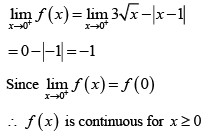Competition Level Test: Continuity And Differentiability- 2 - Question 2

### If the function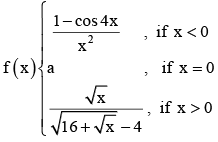is continuous at x = 0 then a =

Detailed Solution for Competition Level Test: Continuity And Differentiability- 2 - Question 2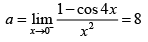Competition Level Test: Continuity And Differentiability- 2 - Question 3

###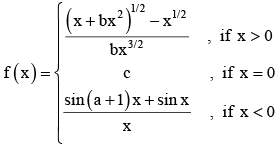is continuous at x = 0 then

Detailed Solution for Competition Level Test: Continuity And Differentiability- 2 - Question 3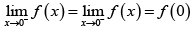Competition Level Test: Continuity And Differentiability- 2 - Question 4

The function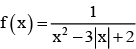is discontinuous at the points

Detailed Solution for Competition Level Test: Continuity And Differentiability- 2 - Question 4

f (x) is discontinuous when x2 - 3|x| + 2 = 0
⇒ |x|2 - 3|x| + 2 = 0 ⇒ |x| = 1, 2

Competition Level Test: Continuity And Differentiability- 2 - Question 5

The values of a and b if f is continuous at x = 0, where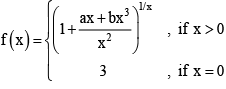Detailed Solution for Competition Level Test: Continuity And Differentiability- 2 - Question 5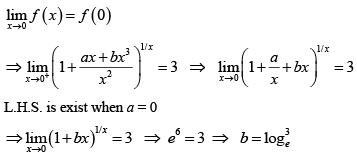Competition Level Test: Continuity And Differentiability- 2 - Question 6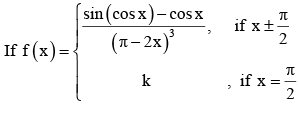is continuous at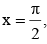then k =

Detailed Solution for Competition Level Test: Continuity And Differentiability- 2 - Question 6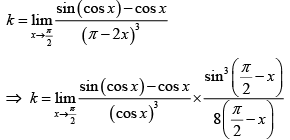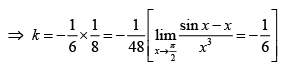Competition Level Test: Continuity And Differentiability- 2 - Question 7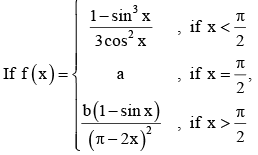so that f(x) is continuous at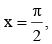then

Detailed Solution for Competition Level Test: Continuity And Differentiability- 2 - Question 7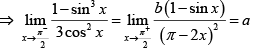By L-Hospital rule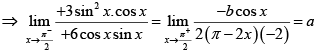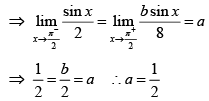Competition Level Test: Continuity And Differentiability- 2 - Question 8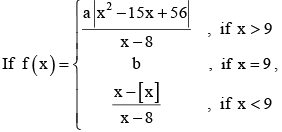where [.] denotes greatest integer function and the function is continuous then

Detailed Solution for Competition Level Test: Continuity And Differentiability- 2 - Question 8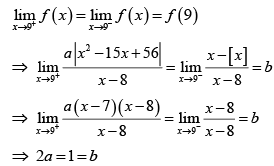Competition Level Test: Continuity And Differentiability- 2 - Question 9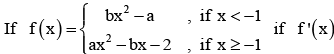is continuous everywhere. Then the  equation whose roots are a and b is

Detailed Solution for Competition Level Test: Continuity And Differentiability- 2 - Question 9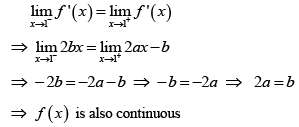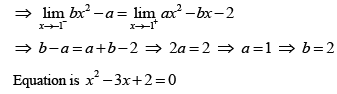Competition Level Test: Continuity And Differentiability- 2 - Question 10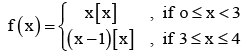where [x] is the greatest integer function. The function f (x) is

Detailed Solution for Competition Level Test: Continuity And Differentiability- 2 - Question 10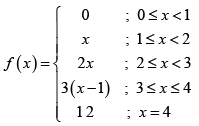Competition Level Test: Continuity And Differentiability- 2 - Question 11

The function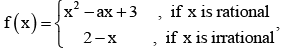is continuous at exactly two  points then the possible values of ' a ' are

Detailed Solution for Competition Level Test: Continuity And Differentiability- 2 - Question 11

f (x) is continuous when x2 - ax + 3 =2 - x
⇒ x2 - a -1 x + 1 = 0. This must have two distinct roots ⇒ Δ > 0 ⇒ (a -1)2 - 4 > 0

Competition Level Test: Continuity And Differentiability- 2 - Question 12

If the function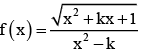is continuous for every x ∈ R then

Detailed Solution for Competition Level Test: Continuity And Differentiability- 2 - Question 12

x2 + kx + 1>0 and x2 - k must not have any real root ;
∴ k2 - 4 < 0 &k < 0
⇒ k ∈ [-2, 2] and k < 0 ⇒ k ∈ [-2, 0)

Competition Level Test: Continuity And Differentiability- 2 - Question 13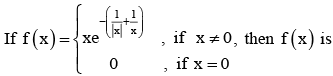Detailed Solution for Competition Level Test: Continuity And Differentiability- 2 - Question 13

|x| is not differentiable at x = 0
|x| is continuous at x = 0

Competition Level Test: Continuity And Differentiability- 2 - Question 14

The function f (x) = cos-1 (cos x) is

Detailed Solution for Competition Level Test: Continuity And Differentiability- 2 - Question 14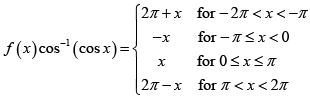f (x) is continuous at x = π, - π

Competition Level Test: Continuity And Differentiability- 2 - Question 15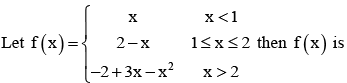Detailed Solution for Competition Level Test: Continuity And Differentiability- 2 - Question 15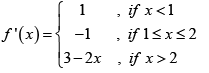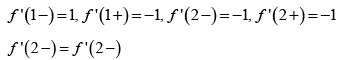Competition Level Test: Continuity And Differentiability- 2 - Question 16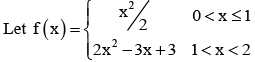then which is correct

Detailed Solution for Competition Level Test: Continuity And Differentiability- 2 - Question 16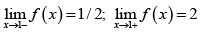Competition Level Test: Continuity And Differentiability- 2 - Question 17

Let f (x) = |x - 1| + |x + 1|

Detailed Solution for Competition Level Test: Continuity And Differentiability- 2 - Question 17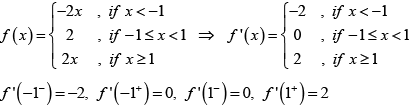Competition Level Test: Continuity And Differentiability- 2 - Question 18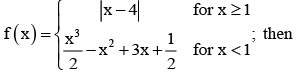Detailed Solution for Competition Level Test: Continuity And Differentiability- 2 - Question 18

Since g(x) = |x| is a continuous function and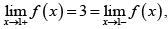so f is continuous function. In particular f is continuous at a = 1 and x = 4) f is clearly not differentiable at x = 4) Since g(x) = |x| is not differentiable at x = 0. Now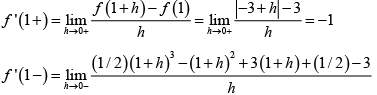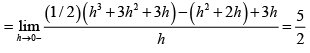Competition Level Test: Continuity And Differentiability- 2 - Question 19

The set of all points where the function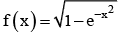is differentiable is

Detailed Solution for Competition Level Test: Continuity And Differentiability- 2 - Question 19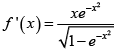is not differentiable only at x = 0

Competition Level Test: Continuity And Differentiability- 2 - Question 20

If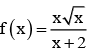then derivative of f(x) at x = 0 is

Detailed Solution for Competition Level Test: Continuity And Differentiability- 2 - Question 20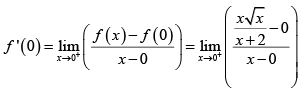Competition Level Test: Continuity And Differentiability- 2 - Question 21

If f : R → R be a differentiable function, such that f (x + 2y) = f (x) + f (2y) + 4xy for all x, y ∈ R then

Detailed Solution for Competition Level Test: Continuity And Differentiability- 2 - Question 21

f (x + 2y) = f (x) + f (2 y) + 4xy for x, y ∈ R putting x = y = 0, we get f (0) = 0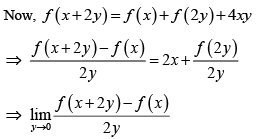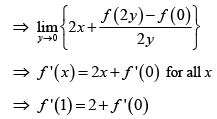Competition Level Test: Continuity And Differentiability- 2 - Question 22

Let f be a differentiable function satisfying the condition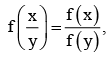for all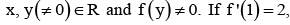, then f ' (x) is equal to

Detailed Solution for Competition Level Test: Continuity And Differentiability- 2 - Question 22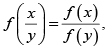replacing x and y both by 1, we get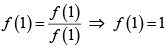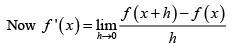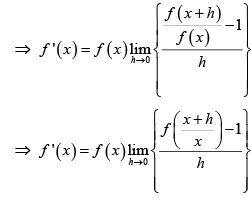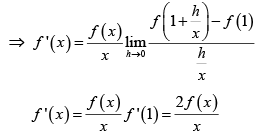Competition Level Test: Continuity And Differentiability- 2 - Question 23

The function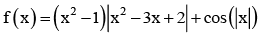is not differentiable at

Detailed Solution for Competition Level Test: Continuity And Differentiability- 2 - Question 23

By verification f ' (2 -) ≠ f ' (2 +)
∴ f(x) is not differentiable at x = 2

Competition Level Test: Continuity And Differentiability- 2 - Question 24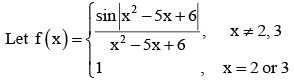then set of all points where f is differentiable is

Detailed Solution for Competition Level Test: Continuity And Differentiability- 2 - Question 24

The function is clearly differentiable except possible at x = 2, 3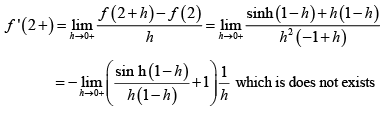Competition Level Test: Continuity And Differentiability- 2 - Question 25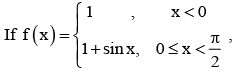Competition Level Test: Continuity And Differentiability- 2 - Question 26

Let h(x) = min {x, x2} for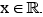Then which of the following is correct

Detailed Solution for Competition Level Test: Continuity And Differentiability- 2 - Question 26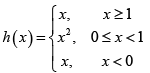From the graph it is clear that h is continuous. Also h is differentiable except possible at x = 0 & 1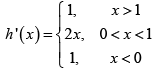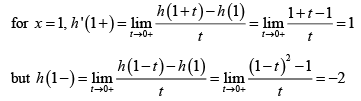so h is not differentiable at 1
similarly h' (0 +)= 0 but h ' (0 - ) = 1

Competition Level Test: Continuity And Differentiability- 2 - Question 27

If f (x + y) = 2f (x) f (y) for all x, y ∈ R where f ' (0) = 3 and f (4) = 2, then f ' (4) is equal to

Detailed Solution for Competition Level Test: Continuity And Differentiability- 2 - Question 27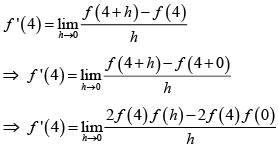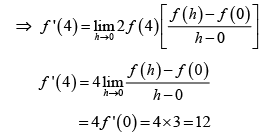Competition Level Test: Continuity And Differentiability- 2 - Question 28

If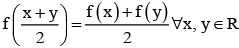and f ' (0) = -1, f (0) = 1, then f (2) =

Detailed Solution for Competition Level Test: Continuity And Differentiability- 2 - Question 28

Take  f (x) = ax+ b

Competition Level Test: Continuity And Differentiability- 2 - Question 29

Let f (x) be differentiable function such that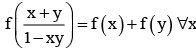and y. If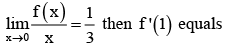Detailed Solution for Competition Level Test: Continuity And Differentiability- 2 - Question 29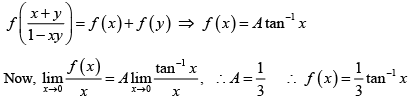Competition Level Test: Continuity And Differentiability- 2 - Question 30

Let f : R → R be a function defined by f (x) = min {x + 1, |x| + 1}, Then which of the following is true?

Detailed Solution for Competition Level Test: Continuity And Differentiability- 2 - Question 30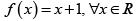## Mathematics (Maths) Class 12

209 videos|218 docs|139 tests
Information about Competition Level Test: Continuity And Differentiability- 2 Page
In this test you can find the Exam questions for Competition Level Test: Continuity And Differentiability- 2 solved & explained in the simplest way possible. Besides giving Questions and answers for Competition Level Test: Continuity And Differentiability- 2, EduRev gives you an ample number of Online tests for practice

## Mathematics (Maths) Class 12

209 videos|218 docs|139 tests(Scan QR code)